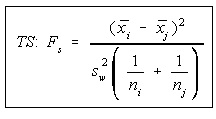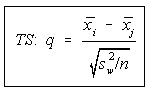#### Scheffe' and Tukey Tests

Scheffe' and Tukey Tests:

Whenever the decision from One-Way Analysis of Variance is to refuse the null hypothesis, it signifies that at least one of the means is not similar as the other means. What we require is a way to figure out where the differences lie, not just that there is the difference.

This is where the Tukey and Scheffe' tests come to play. They will assist us in analyzing pairs of means to see when there is a difference -- much similar to the difference of two means.

Hypotheses:

Both the tests are set up to test when pairs of means are distinct. The formulas refer to mean i and mean j. The values of i and j differ and the total number of tests will be equivalent to the combination of k objects, 2 at a time C(k,2), where k is number of samples.

Ho: μi = μj
H1: μi ≠μj

Scheffé Test:

The Scheffe' test is customarily employed with unequal sample sizes, though it could be employed with equivalent sample sizes.

The critical value for Scheffe' test is the degree of freedom for between variance times the critical value for one-way ANOVA. This can be simplified as:

CV = (k-1) F(k-1,N-k,alpha)

The test statistic is a little bit tough to calculate. Pure mathematicians will state that this shouldn't be termed F as it doesn't encompass an F distribution (it is the degree of freedom times an F), however we will live with it.Discard H0 if the test statistic is bigger than the critical value. Note that this is a right tail test. When there is no difference between the means, numerator will be close to zero, and therefore executing a left tail test wouldn't illustrate anything.

Tukey Test:

The Tukey test is usable only if the sample sizes are similar.

The Critical Value is looked-up in a table. This is Table N in Bluman text. There are really some different tables, one for each and every level of significance. The number of samples k, is employed as an index all along the top, and the degree of freedom for within group variance, v = N - k, are employed as an index all along the left side.The test statistic is found out by dividing the difference among the means by the square root of ratio of the within group variation and sample size.

Refuse the null hypothesis when the absolute value of test statistic is bigger than the critical value (just similar to the linear correlation coefficient critical values).

Latest technology based Statistics Online Tutoring Assistance

Tutors, at the www.tutorsglobe.com, take pledge to provide full satisfaction and assurance in Statistics help via online tutoring. Students are getting 100% satisfaction by online tutors across the globe. Here you can get homework help for Statistics, project ideas and tutorials. We provide email based Statistics help. You can join us to ask queries 24x7 with live, experienced and qualified online tutors specialized in Statistics. Through Online Tutoring, you would be able to complete your homework or assignments at your home. Tutors at the TutorsGlobe are committed to provide the best quality online tutoring assistance for Statistics Homework help and assignment help services. They use their experience, as they have solved thousands of the Statistics assignments, which may help you to solve your complex issues of Statistics. TutorsGlobe assure for the best quality compliance to your homework. Compromise with quality is not in our dictionary. If we feel that we are not able to provide the homework help as per the deadline or given instruction by the student, we refund the money of the student without any delay.